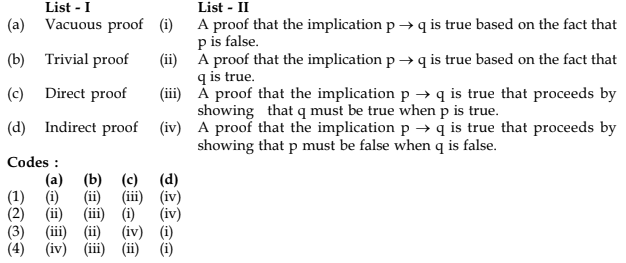Skip to content
Related Articles
UGC-NET | UGC NET CS 2015 Dec – II | Question 8
• Last Updated : 01 May, 2018

Match the following terms:(A) (1)
(B) (2)
(C) (3)
(D) (4)

Answer: (A)

Explanation:

• Vacuous proof is a proof in which the implication p → q is true based on the fact that p is false.
• Trivial proof is a proof in which the implication p → q is true based on the fact that q is true.
• Direct proof is A proof in which the implication p → q is true that proceeds by showing that q must be true when p is true.
• Indirect proof a proof in which the implication p → q is true that proceeds by showing that p must be false when q is false.

So, option (A) is correct.

Quiz of this Question

My Personal Notes arrow_drop_up